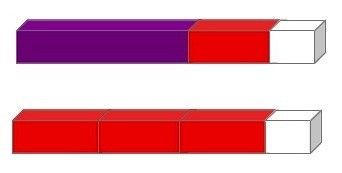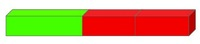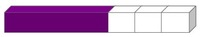# Using lengths to represent equality

Alignments to Content Standards: 1.OA.D.7

#### Materials

Cuisenaire rods or paper strips cut to whole centimeter lengths

#### Actions

Students work in pairs.

• One student puts a few rods (or strips) end-to-end.

• The other student matches that length with a different combination of rods (or strips).

• When two different ways of making the same length are found, the students write a number sentence reflecting the equality.

For example, if the first student uses a rod of length 4, a rod of length 2 and a rod of length 1 and the second student uses three rods of length 2 and a rod of length 1 (as shown below), they should write $4+2+1=2+2+2+1.$$${\Large 4+2+1=2+2+2+1}$$

## IM Commentary

• The act of trying to find equal lengths with the rods helps students develop a physical understanding for the meaning of equality. Students are more likely to generate and understand complex equalities than they would be able to do only abstractly.

• A variation that would help scaffold students from physical objects to drawings would be to have them draw a color-matched picture of their Cuisenaire rods on graph paper in addition to writing the equation. For example, a student might draw the following picture for the example given in the task statement:• One variation may include having the teacher choose one (larger) Cuisenaire rod. Then each student in each pair would match that length. From there, the students write a number sentence describing an equality based on the rods they chose. This variation connects to 1.MD.1 and 1.MD.2 as well as 1.OA.7. Some students may need a small white board or paper to record how they made their long rod as they create it; otherwise some students might forget which rods they chose.

## Solution

For example, if the first student arranges Cuisenaire end to end like this (in this example there are 3 rods)then the second student could use Cuisenaire rods to match the total length like thisThe two students then write a number sentence to describe the relationship. $$\large{3+2+2=4+1+1+1}$$